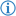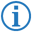The less than operator is a type of comparison operator which returns the Boolean value `true` if the first number object's value is smaller than the second's, else it returns `false`.

Its token is named LESS_THAN.

## FDS### Example

`If`` ``Spike's age`` ``is less than`` ``23`` ``then``:` `...`

This executes the next part of code only if the variable `Spike's age` is smaller than `23`.

This is equivalent to the following Java code:

```if(SpikesAge<10) { ...
```